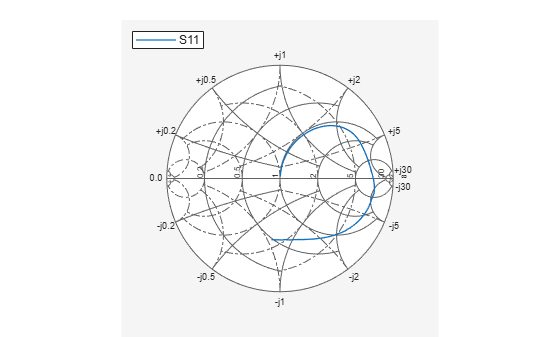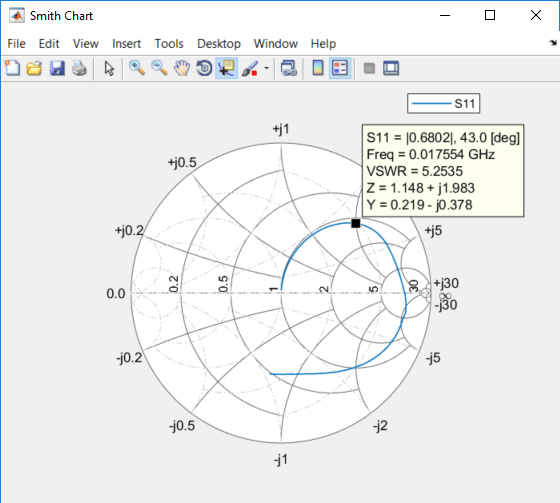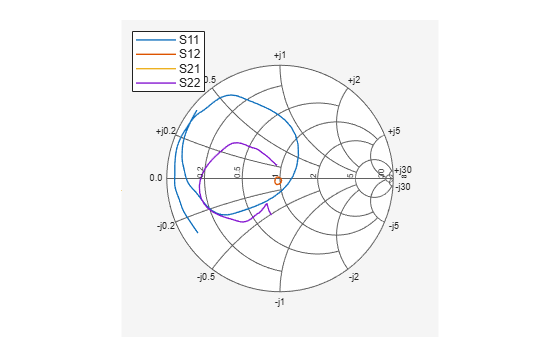Documentation

### This is machine translation

Mouseover text to see original. Click the button below to return to the English version of the page.

To view all translated materials including this page, select Country from the country navigator on the bottom of this page.

# smithplot

Plot measurement data on Smith chart

## Syntax

``smithplot(data)``
``smithplot(frequency,data)``
``smithplot(ax,___)``
``smithplot(hnet)``
``smithplot(hnet,i,j)``
``smithplot(hnet,[i1,j1;i2,j2;....,in,jn])``
``s = smithplot(___)``
``s = smithplot('gco')``
``smithplot(___,Name,Value)``

## Description

example

````smithplot(data)` creates a Smith chart based on input data values. NoteThe Smith chart is commonly used to display the relationship between a reflection coefficient, typically given as S11 or S22, and a normalized impedance. ```
````smithplot(frequency,data)` creates a Smith chart based on frequency and data values.```
````smithplot(ax,___)` creates a Smith chart with a user defined axes handle, `ax`, instead of the current axes handle. Axes handles are not supported for network parameter objects. This parameter can be used with either of the two previous syntaxes.```
````smithplot(hnet)` plots all the network parameter objects in `hnet`.```

example

````smithplot(hnet,i,j)` plots the (i,j)th parameter of `hnet`. `hnet` can be a network parameter, an rfckt, an rfdata, an nport, or an rfbudget object.```

example

````smithplot(hnet,[i1,j1;i2,j2;....,in,jn])` plots multiple parameters (i1, j1, i2, j2, …, in, jn) of `hnet`. `hnet` can be a network parameter, an rfckt, an rfdata, an nport, or an rfbudget object. NoteFor rfbudget objects, smith plot is restricted to reflection coefficients. ```
````s = smithplot(___)` returns a Smith chart object handle so you can customize the plot and add measurements. ```
````s = smithplot('gco')` returns a Smith chart object handle of the current plot. This syntax is useful when the function handle, `p` was not returned or retained.```
````smithplot(___,Name,Value)` creates a Smith chart with additional properties specified by one or more name-value pair arguments. `Name` is the property name and `Value` is the corresponding property value. You can specify several name-value pair arguments in any order as `Name1`, `Value1`, `...`, `NameN`, `ValueN`. Properties not specified retain their default values.```

## Examples

collapse all

Plot the Smith plot of s-parameters data file, `passive.s2p`.

`data = nport('passive.s2p')`
```data = nport: N-port element NetworkData: [1x1 sparameters] Name: 'Sparams' NumPorts: 2 Terminals: {'p1+' 'p2+' 'p1-' 'p2-'} ```
`smithplot(data);`Plot the Smith plot of S11 of s-parameter data file using an impedance of 75 ohms.

```data = sparameters('passive.s2p' ); s = sparameters(data,75); p = smithplot(s,1,1, 'GridType','ZY');```Use the data cursor icon in the toolbar to insert a cursor on your smith plot chart. You now know the S11, VSWR, Impedance, and frequency values at that cursor. For admittance value, change the Grid Type.Plot the Smith chart of an rfckt.amplifier object.

```S = read(rfckt.amplifier,'passive.s2p'); ports = [1,1;1,2]; s = smithplot(S,ports); s.LineStyle = {'--', '-'}; ```Use the Smith plot interactive menu for changing line and marker styles.

Plot the Smith plot of s-parameters data file, `default.s2p`.

`data = nport('default.s2p')`
```data = nport: N-port element NetworkData: [1x1 sparameters] Name: 'Sparams' NumPorts: 2 Terminals: {'p1+' 'p2+' 'p1-' 'p2-'} ```
`smithplot(data)`Right click on the S11 line to reveal interactive menu, `DATASET 1. `Use LineStyle to change the style of S11 line on the Smith plot.## Input Arguments

collapse all

Input data, specified as a complex vector or complex matrix.

For a matrix D, the columns of D are independent data sets. For N-by-D arrays, dimensions 2 and greater are independent data sets.

Data Types: `double`
Complex Number Support: Yes

Frequency data, specified as a real vector.

Data Types: `double`

Input objects, specified as one of the following:

• RF Toolbox™ network parameter object

• rfckt object

• rfdata object

• nport object

• rfbudget object.

Data Types: `double`

## Output Arguments

collapse all

Smith chart object handle. You can use the handle to customize the plot and add measurements using MATLAB® commands.

## Tips

• To list all the property `Name,Value` pairs in `smithplot`, use `details(s)`. You can use the properties to extract any data from the Smith chart. For example, `s = smithplot(data,'GridType','Z')` displays the impedance data grid from the Smith chart.

• For a list of properties of `smithplot`, see SmithPlot Properties.

• You can use the `smithplot` interactive menu to change the line and marker styles.